# Bayesian model selection

```Tom Minka
```Bayesian model selection uses the rules of probability theory to select among different hypotheses. It is completely analogous to Bayesian classification. It automatically encodes a preference for simpler, more constrained models, as illustrated at right. Simple models, e.g. linear regression, only fit a small fraction of data sets. But they assign correspondingly higher probability to those data sets. Flexible models spread themselves out more thinly.

The probability of the data given the model is computed by integrating over the unknown parameter values in that model:which reduces to a problem of calculus. This quantity is called the evidence for model M. Specific examples of computing the evidence follow.

A useful property of Bayesian model selection is that it is guaranteed to select the right model, if there is one, as the size of the dataset grows to infinity.

## Polynomial fitting

A central issue in polynomial fitting is selecting the degree of the polynomial. To do this, we compute the probability of the data for each possible degree. For a given degree, this requires integrating over all possible polynomial fits, which can be done analytically. The results are shown below.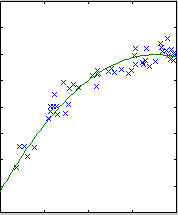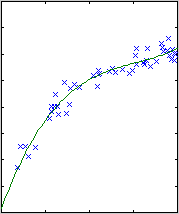degree 1 degree 2 degree 3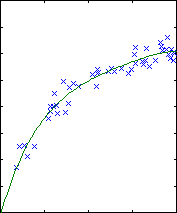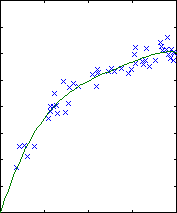degree 4 degree 5 degree 6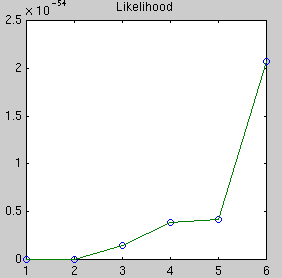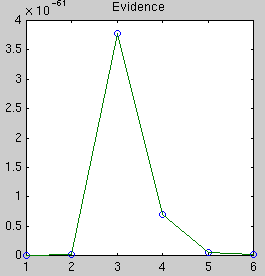The likelihood only considers the single most probable curve, as shown for each degree. The likelihood is the negative squared-error of the fit. It always increases with increasing degree. But the evidence reaches a maximum at degree 3, which happens to be the true degree in this case. The data is much more likely to have arisen from a third-degree polynomial than a high-degree polynomial which just happened to look third-degree.

The same procedure works for regression with splines, radial basis functions, and feedforward neural networks. See my paper "Bayesian linear regression".

## Changepoint analysis

A changepoint model allows different parts of a dataset to obey different probability laws. For example, all data received before time t follows model A, while data received after time t follows model B. A central issue is determining the number of changepoints. The likelihood is not sufficient for this purpose because it will always prefer more changepoints. We can use Bayesian model selection by computing the probability of the data for each number of changepoints. For each number of changepoints, we need to integrate over all possible changepoint positions and all sub-models given those changepoints. This technique is described in my paper "Bayesian linear regression".

This is actually quite simple mathematically since the integral over changepoint positions is a discrete sum. For example, consider a linear regression changepoint model. The integral over possible lines was solved in the curve fitting problem. Now we sum that quantity over possible changepoints. Here are two examples: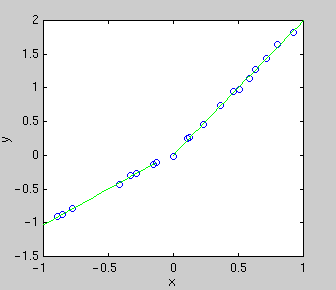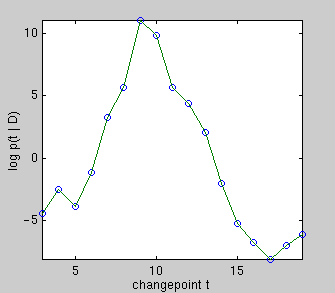Evidence for each changepoint Evidence for each changepoint

On the left, there really is a changepoint at t = 9. Plotted underneath is the evidence for each changepoint position, which is an integral over the parameters of the two linear sub-models. Summing over all changepoint positions gives the probability of the data given that there is one changepoint. Comparing this to the evidence for zero changepoints, i.e. the evidence for a single line, gives an overwhelming probability of there being a changepoint.

On the right, there really is no changepoint. Summing over all changepoint positions gives a probability lower than that of a single line. So we correctly infer that there is no changepoint.

## Principal component analysis

Principal component analysis (PCA) decomposes high-dimensional data into a low-dimensional subspace component and a noise component. This decomposition is useful for data compression as well as de-noising, making it a common first step for many data processing tasks. Here we use PCA for density estimation, or equivalently lossless coding.

A central issue in PCA is choosing the dimensionality of the subspace component so that all of the noise, but none of the signal, is removed. To do this, we compute the probability of the data for each possible subspace dimensionality. For a given dimensionality, this requires integrating over all possible PCA decompositions, i.e. all possible subspaces. This can be done analytically, but it is simpler to approximate it using Laplace's method. Laplace's method essentially just measures the sensitivity of the likelihood to each parameter (the parameters here are the basis vectors for the subspace). Even simpler is the BIC method, which assumes the same sensitivity to each parameter. Both are correct asymptotically.

In the first example, a synthetic data set of 60 points in 100 dimensions has been sampled. Below is plotted the eigenvalues of the true covariance matrix and the observed covariance matrix. These measure the variance in each dimension. The dimensions after 5 are good candidates for noise, since they all have the same variance. The presence of a signal always increases the variance above the noise floor, which we assume is constant.

 True eigenvalues Observed eigenvalues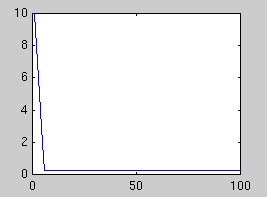Next we plot the evidence for each subspace dimensionality, using Laplace's method and BIC:

 Likelihood Laplace evidence BIC evidence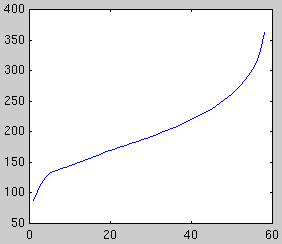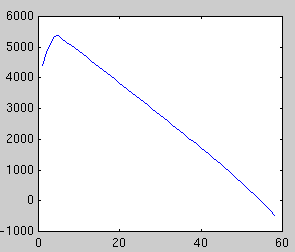Both methods peak at dimensionality 5, however BIC is increasingly unreliable for large dimensionalities. In some cases, the second peak may exceed the first.

Cross-validation, a non-Bayesian model selection technique, also picks 5. However it is far more costly to compute. The evidence approximations can be computed directly from the eigenvalue spectrum and they are very fast. See the paper "Automatic choice of dimensionality for PCA".

## Classification

In classification we want to construct a function which takes in a measurement and outputs c when the measurement is most likely to be in class c. In other words, we want to construct boundaries in measurement space which delineate the classes. Once we have chosen a parametric form for the function, it is straightforward to find the function which best fits training data. But there are many different parametric forms to choose from and so we have a model selection problem.

If the classifier function is linear in the parameters, then the task becomes similar to curve fitting as above. We still have the choice of what basis functions to use. Consider three different bases: linear, quadratic, and Gaussian kernel. Linear means our classifier function is simply a weighted sum of the measurements---the boundary is a line. Quadratic means the function is a quadratic function of the measurements---the boundary is a parabola, hyperbola, or ellipse. Gaussian kernel means our classifer function is a weighted sum of Gaussians centered on the data points. Example classifiers are shown below.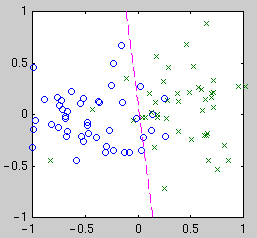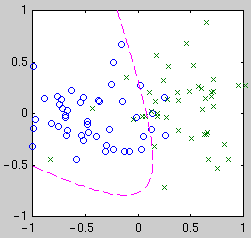Linear Quadratic Gaussian kernel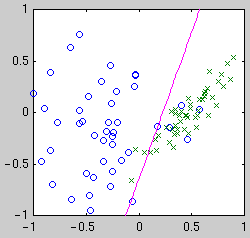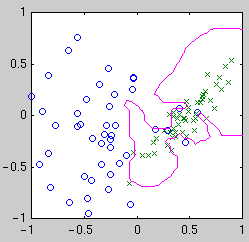Linear Quadratic Gaussian kernel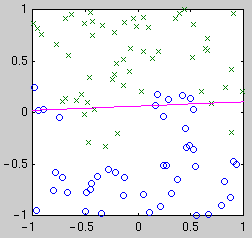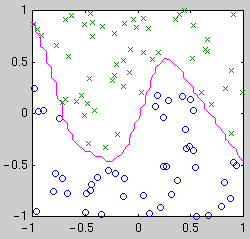Linear Quadratic Gaussian kernel

Intuitively, we see that the "best" classifier in each case balances simplicity against the need to fit the data. For Bayesian model selection, we integrate over the possible classifier functions for each model. Performing this integral is complicated by the fact that the likelihood of a classifier function is not a smooth function of its parameters. The likelihood is relatively constant until a data point becomes mislabeled, when it goes down abruptly. Thus the integral has a combinatorial flavor.

In my PhD work, I developed a deterministic method for approximating this integral which is faster than Monte Carlo methods. See "Expectation Propagation for approximate Bayesian inference".

## Other problems

Bayesian model selection is useful for many other problems, such as choosing the size of a mixture model or the structure of a Hidden Markov Model. It has the potential to become a standard part of every analyst's toolbox.